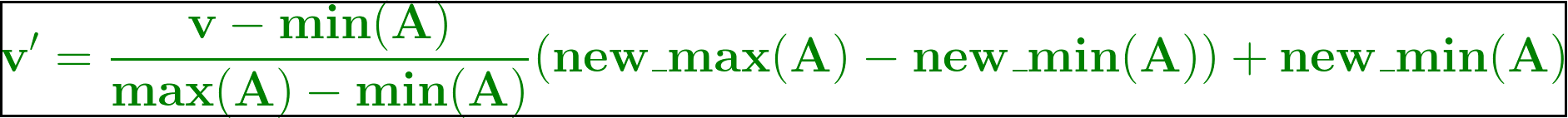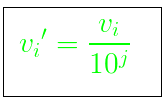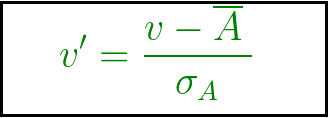Open in App
Not now

# What is Data Normalization?

• Last Updated : 18 Aug, 2021

Normalization is a pre-processing stage of any type of problem statement. In particular, normalization takes an important role in the field of soft computing, cloud computing, etc. for manipulation of data, scaling down, or scaling up the range of data before it becomes used for further stages. There are so many normalization techniques there, namely Min-Max normalization, Z-score normalization, and Decimal scaling normalization.

Normalization is scaling the data to be analyzed to a specific range such as [0.0, 1.0] to provide better results.

### What is Data Normalization?

Data transformation operations, such as normalization and aggregation, are additional data preprocessing procedures that would contribute toward the success of the data extract process.

Data normalization consists of remodeling numeric columns to a standard scale. Data normalization is generally considered the development of clean data. Diving deeper, however, the meaning or goal of data normalization is twofold:

• Data normalization is the organization of data to appear similar across all records and fields.
• It increases the cohesion of entry types, leading to cleansing, lead generation, segmentation, and higher quality data.

### Importance of Data Normalization

Data Normalization disposes of various anomalies that can make an examination of the information more complicated. A portion of those irregularities can manifest from erasing information, embedding more data, or refreshing existing data. Once those mistakes are worked out and eliminated from the framework, further advantages can be acquired through different jobs in the data and data examination.

It is for the most part through data normalization that the data inside a data set can be designed so that it can be visualized and examined.

• We can have more clustered indexes.
• Index searching is often faster.
• Data modification commands are faster.
• Fewer null values and less redundant data, making your data more compact.
• Data modification anomalies are reduced.
• Normalization is conceptually cleaner and easier to maintain and change as your needs change.
• Searching, sorting, and creating indexes is faster, since tables are narrower, and more rows fit on a data page.

### Need of Normalization

Normalization is generally required when we are dealing with attributes on a different scale, otherwise, it may lead to a dilution in the effectiveness of an important equally important attribute(on a lower scale) because of other attributes having values on a larger scale. In simple words, when multiple attributes are there but attributes have values on different scales, this may lead to poor data models while performing data mining operations. So they are normalized to bring all the attributes on the same scale.

### Data Normalization Methods

Normalization is a scaling technique or a mapping technique or a pre-processing stage. Where we can find a new range from an existing one. It can be helpful for prediction or forecasting purposes a lot. As we know, there are so many ways to predict or forecast, but all can vary with each other a lot. So, to maintain the large variety of prediction and forecasting predictions, normalization techniques are required to make them closer. There are some existing normalization techniques as mentioned below:

Min-Max normalization: In this technique of data normalization, a linear transformation is performed on the original data. The minimum and maximum value from data are fetched and each value is replaced according to the following formula.Where A is the attribute data,
Min(A), Max(A) are the minimum and maximum absolute values of A respectively.
v’ is the new value of each entry in data.
v is the old value of each entry in data.
new_max(A), new_min(A) is the max and min values of the range(i.e boundary value of range required) respectively.

Normalization by decimal scaling: It normalizes by moving the decimal point of values of the data. To normalize the data by this technique, we divide each value of the data by the maximum absolute value of the data. The data value, vi, of data, is normalized to vi by using the formula below :where j is the smallest integer such that max(|vi‘|)<1.

Z-score normalization or Zero mean normalization: In this technique, values are normalized based on mean and standard deviation of the data A. The formula used is:v’, v is new and old of each entry in data respectively. σA, A is the standard deviation and mean of A respectively.

My Personal Notes arrow_drop_up